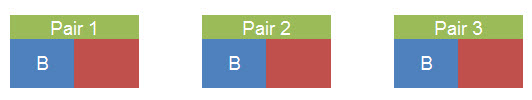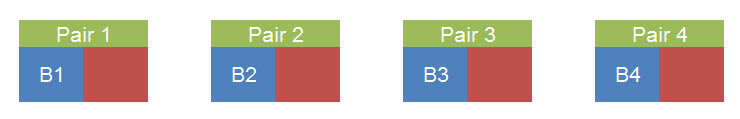Probability problem 1

Question (Probability practice problems): A survey conducted in a particular college yielded the following data.

42% had their own vehicle

39% had a job

22% had a vehicle and job

10% only had job

4% had vehicle, job and played basketball.

a) Calculate what percentage only played basketball

b) Calculate the percentage of students who do not have vehicle, job or play basketball

Show Solution

Solution:

Draw a Venn diagram

Now rewrite given information in terms of a,b,c,d,e,f,g

a – Only vehicle  b – Only basketball  c – Only job  d,e,f represent intersections and g represents intersection of all three parameters

1. a+d+e+g = 42
2. b+d+g+f = 48
3. c+f+g+e = 39
4. g+e = 22
5. d+g = 16
6. c = 10
7. g = 4
8. e = 18 (from 4)
9. d = 12 (from 5)
10. a = 8 (from 1,4,9)
11. f = 7 (from 3,4,6)
12. b = 25 (from 2,5,11)

a)Only basketball= b = 25%

b)No Vehicle, no job, no basketball= 1 – (a+b+c+d+e+f+g) = 16%

Probability problem 2

Question (Probability practice problems):

In how many ways letters of the word ‘ENCYCLOPEDIA’ be rearranged such that all the vowels are together?

What is the probability that all the vowels come together out of all the possible arrangements of this word?

Show Solution

Solution:

The word ‘ENCYCLOPEDIA’ can be broken down as shown below

Vowels: E, O, E, I, A

Consonants: N, C, Y, C, L, P, D

Step 1:

Vowels should occur together

E, O, E, I, A

Number of rearrangements within Vowels pack: 5! / 2!  = 60 ways  (We divide by 2! as there are 2 ‘E’ in vowels pack)

Step 2:

Now the pack of vowels can be considered as a single entity.

# of consonants = 7

Total entities = 8 ( 7 consonants and 1 vowels pack)

Step 3:

# of ways in which these 8 entities could be rearranged among themselves

=  8! / 2! = 20,160 ways

Step 4:

Total # of desired combinations = 60 * 20,160 = 12, 09, 600 ways

Probability calculation:

Step 1: Total combinations of word ‘ENCYCLOPEDIA’

= 12! / (2!  * 2!) { 2 ‘E’  and 2 ‘C’ } = 119, 750, 400 ways

Step 2: Total desired combinations (Vowels together) = 12, 09, 600 ways

Step 3: Probability that vowels come together = 12,09,600/119,750,400 = 0.01

Probability problem 3

Question (Probability practice problems):

In how many ways 4 boys and 6 girls could be grouped into 3 pairs?

Show Solution

Solution:

Method1:

Our objective is to form 3 pairs.

Each Pair will have one boy. In total, we require 3 boys for 3 pairs.

Step1: Select boys for three pairs

• # of ways of selecting 3 boys out of 4 = 4C3 = 4 ways

Now, we will have to fill the pairs with girls as shown below.Step2: Fill Pair 1 girl

• # of ways of selecting 1 girl out of 6 girls = 6 ways

Step3: Fill Pair 2 girl

• # of ways of selecting 1 girl out of 5 remaining girls = 5 ways

Step4: Fill Pair 3 girl

• # of ways of selecting 1 girl out of 4 remaining girls = 4 ways

Number of ways of filling the 3 pairs = {4}*{6*5*4} = 480 ways

Method 2:

To form 3 pairs, we require 3 boys and 3 girls.

• Step1: # of ways of selecting 3 boys out of 4 = 4C3 = 4 ways
• Step2: # of ways of selecting 3 girls out of 6 = 6C3 = 20 ways
• Step3: # of ways of selecting 3 boys and 3 girls = 4*20 = 80 ways (Why multiply? – Independent events)
• Step4: # of ways of grouping 3 boys and 3 girls into 3 pairs = 3! = 6 ways.
• Step5: Total # of ways = 80*6 = 480 ways

Probability problem 4

Question (Probability practice problems):

There are six girls and five boys who play tennis in a class. If we want to select two boys and two girls for conducting a mixed doubles tennis match, how many such combinations are possible?

Show Solution

Solution:

Our objective is to select two boys and two girls to form two teams (team and an opposite team)

Number of ways of selecting two boys out of 5 = 5C2 = 10

Number of ways of selecting two girls out of 6 = 6C2 = 15

Number of ways of selecting two boys and two girls = 10*15 = 150 ways.

Let us assume the selected boys and girls are B1, B2, G1 and G2.

Number of ways of forming two teams from these four = 2 (How? – {(B1G1, B2G2), (B1G2, B2G1)}

Total number of ways in which the teams can be formed = 150 *2 = 300 ways.

Probability problem 5

Question (Probability practice problems):

In how many ways can four boys and four girls could be grouped into four pairs?

Show Solution

Solution:

Boys – B1, B2, B3, B4

Girls – G1, G2, G3, G4

Our objective is to form four pairs. Remember that we are not concerned with the order of pairs. As we have 4 boys and we need to form 4 pairs, each pair contains 1 boy.

Below are the 4 pairs that we get finally. Now, our objective is to fill the pairs with girls.Pair 1: This can be filled in 4 ways (Select 1 from 4 girls)

Pair 2: This can be filled in 3 ways (Select 1 from remaining 3 girls)

Pair 3:  This can be filled in 2 ways (Select 1 from remaining 2 girls)

Pair 4: This can be filled in only 1 way (Select 1 from remaining 1 girl)

Total ways of filling : 4*3*2*1 = 4! = 24 ways

Probability problem 6

Question (Probability practice problems):

Five boys and five girls are seated in a row at random, what is the probability that all the five boys sit together (Next to each other)?

Show Solution

Solution:

Total arrangements:

Let us look at the total possibilities of arranging 10 people in 10 seats

# of ways of arranging 10 people in 10 seats = 10! (10 factorial)

Desired arrangement:

We want 5 boys to sit together.

Fix the starting position for boys group

Let the seats be S1 to S10 as shown below. Group of boys can be seated together starting with first boy seated at S1 or S2 or S3 or S4 or S5 or S6 as shown below.# of ways in which this starting position could be fixed = 6 ways (S1 to S6)

Arrange five boys in the selected five positions

# of ways in which 5 boys could be arranged in 5 positions = 5! (5 Factorial)

Arrange five girls in the remaining five positions

# of ways in which 5 girls could be arranged in 5 positions = 5! (5 Factorial)

Total number of desired arrangements:

Total # of desired arrangements = 6*5!*5!

Probability of desired arrangement

= (Total # of desired arrangements / Total # of arrangements)

= (6*5!*5!)/10! = 1/42